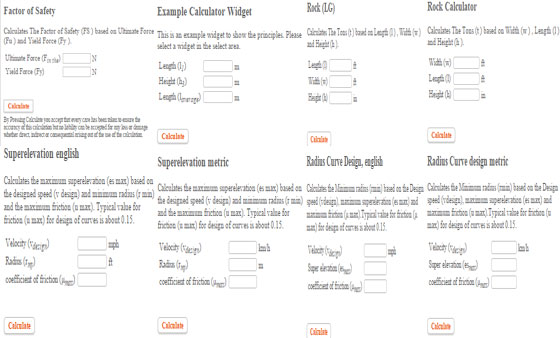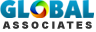# Free Civil Engineering Calculator Widgets

A collection of free online civil engineering calculators Widgets used for surveying and designing of highways and roadways Construction. Showing widget one to eight matching Widgets:

Factor of Safety: This Calculator Widget can be described as a widget that Calculates The Factor of Safety (FS) based on Ultimate Force (Fu) and Yield Force (Fy).

Top Soil: This Calculator Widget can be described as a widget that Calculates The Tons (t) based on Length (l) , Width (w) and Height (h).

Rock (LG): This Calculator Widget can be described as a widget that Calculates The Tons (t) based on Length (l) , Width (w) and Height (h).

Rock Calculator: Calculates The Tons (t) based on Width (w) , Length (l) and Height (h).

Superelevation english: Calculates the maximum superelevation (es max) based on the designed speed (v design) and minimum radius (r min) and the maximum friction (u max). Typical value for friction (u max) for design of curves is about 0.15.

Superelevation metric: Calculates the maximum superelevation (es max) based on the designed speed (v design) and minimum radius (r min) and the maximum friction (u max). Typical value for friction (u max) for design of curves is about 0.15.

Radius Curve Design, english:: Calculates the Minimum radius (rmin) based on the Design speed (vdesign), maximum superelevation (es max) and maximum friction (µ max).Typical value for friction (µ max) for design of curves is about 0.15.

Radius Curve design metric:: Calculates the Minimum radius (rmin) based on the Design speed (vdesign), maximum superelevation (es max) and maximum friction (u max).Typical value for friction (u max) for design of curves is about 0.15.# Takeoff Tools